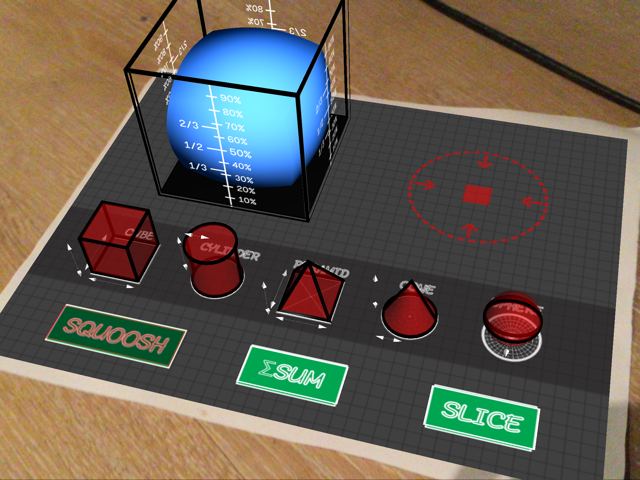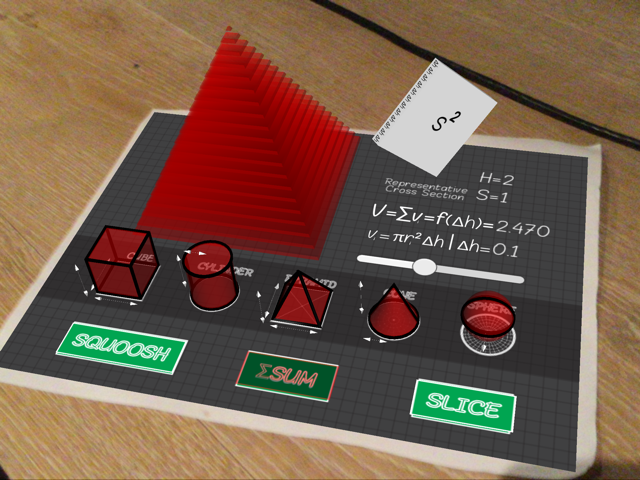## Squoosh Sum Slice Volumes

This module addresses Common Core 8.G.C.9.

This "lab-style" module helps students deduce and intuitively understand the formulae for volumes of prisms, and pyramids, cones, and spheres. Select each corresponding volume to find out more about them.

• Squoosh Lab lets students "squoosh" or compress different volumes each into a unit cube. Students can then see the percentage of an object's volume to the cube's. (This was inspired by a similar Flash-based 2D module via the Annenberg CPB.)A sphere gets squooshed into a rectangular prism. What percentage of the cube is the sphere's compressed volume?

• Sum Lab lets students "slice up" each volume into smaller and smaller slices, to approximate their volumes numerically.A pyramid gets sliced into N tiny slices - the thinner the slices are, the closer we get to the pyramid's volume formula value!

• [Under Development] Slice Lab lets students observe corresponding 2D geometry that form from slicing each volume at different locations.

• [Under Development] Drills - students can see 3D augmented reality versions of everyday objects such as soda cans and baseball's and calculate their volumes.